Diffusion

Diffusion is the process behind Brownian motion. It was described by Albert Einstein in one of his annus mirabilis papers of 1905. What follows applies to homogeneous systems, see diffusion at interfaces for a non-homogeneous case.

The diffusion equation that describes this process is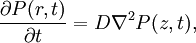$\frac{\partial P(r,t)}{\partial t}= D \nabla^2 P(z,t),$

where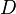$D$ is the (self-)diffusion coefficient. For initial conditions for a Dirac delta function at the origin, and boundary conditions that force the vanishing of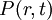$P(r,t)$ and its gradient at large distances, the solution factorizes as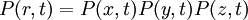$P(r,t)=P(x,t)P(y,t)P(z,t)$, with a spreading Gaussian for each of the Cartesian components: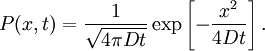$P(x,t)=\frac{1}{\sqrt{4\pi D t}} \exp \left[ - \frac{x^2}{4 D t} \right].$

Einstein relation

It follows from the previous equation that, for each of the Cartesian components, e.g.$x$: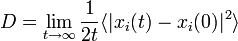$D = \lim_{t \rightarrow \infty} \frac{1}{2t} \langle \vert x_i(t) - x_i(0) \vert^2\rangle$,

for every particle$i$. Therefore, an average over all particles can be employed in order to improve statistics. The same applies to time averaging: in equilibrium the average from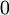$0$ to$t$ must equal the average from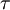$\tau$ to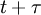$t+\tau$, so several time segments from the same simulation may be averaged for a given interval . Adding all components, the following also applies: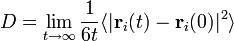$D = \lim_{t \rightarrow \infty} \frac{1}{6t } \langle \vert \mathbf{r}_i(t) - \mathbf{r}_i(0) \vert^2\rangle$

Green-Kubo relation

Main article: Green-Kubo relations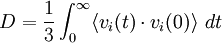$D = \frac{1}{3} \int_0^\infty \langle v_i(t) \cdot v_i(0)\rangle ~dt$

where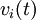$v_i(t)$ is the center of mass velocity of molecule$i$. Note that this connect the diffusion coefficient with the velocity autocorrelation.